Скачать презентацию Mechanical waves By the end of this lecture

l_19_mechanical_waves.ppt

• Размер: 2.9 Mегабайта
• Количество слайдов: 13

## Описание презентации Mechanical waves By the end of this lecture по слайдам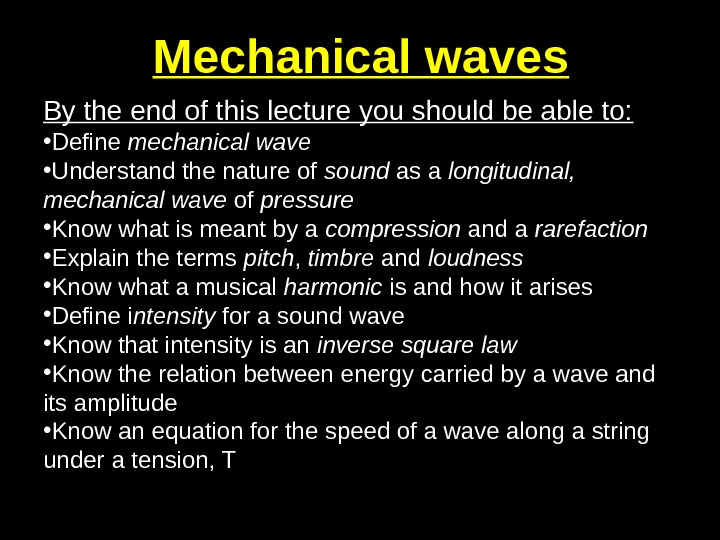Mechanical waves By the end of this lecture you should be able to: • Define mechanical wave • Understand the nature of sound as a longitudinal, mechanical wave of pressure • Know what is meant by a compression and a rarefaction • Explain the terms pitch , timbre and loudness • Know what a musical harmonic is and how it arises • Define i ntensity for a sound wave • Know that intensity is an inverse square law • Know the relation between energy carried by a wave and its amplitude • Know an equation for the speed of a wave along a string under a tension, T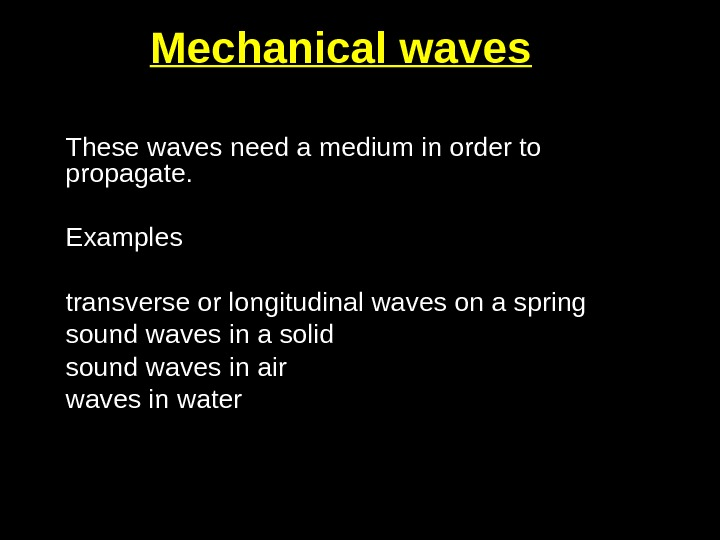Mechanical waves These waves need a medium in order to propagate. Examples transverse or longitudinal waves on a spring sound waves in a solid sound waves in air waves in water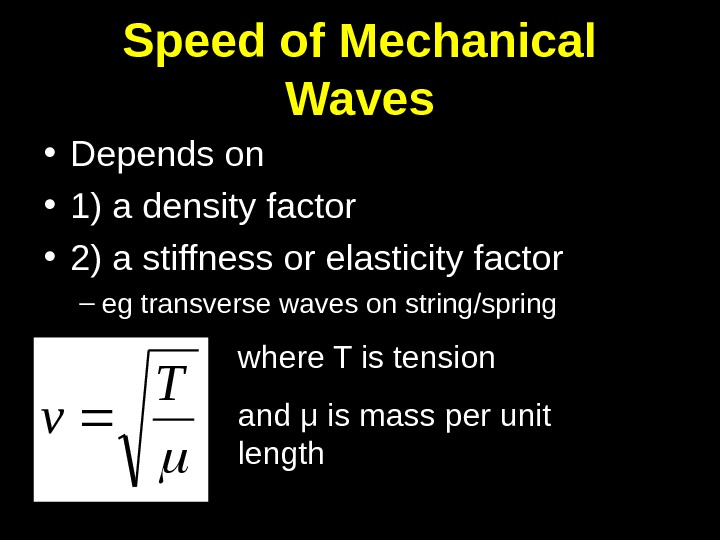Speed of Mechanical Waves • Depends on • 1) a density factor • 2) a stiffness or elasticity factor – eg transverse waves on string/spring T v where T is tension and μ is mass per unit length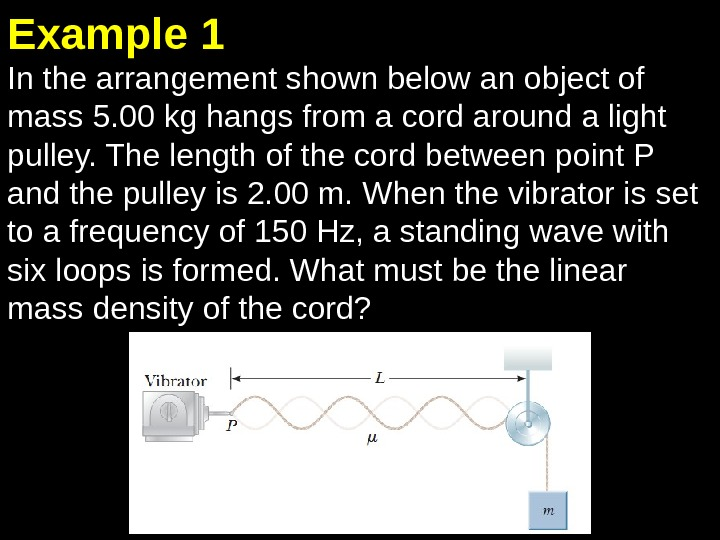In the arrangement shown below an object of mass 5. 00 kg hangs from a cord around a light pulley. The length of the cord between point P and the pulley is 2. 00 m. When the vibrator is set to a frequency of 150 Hz, a standing wave with six loops is formed. What must be the linear mass density of the cord? Example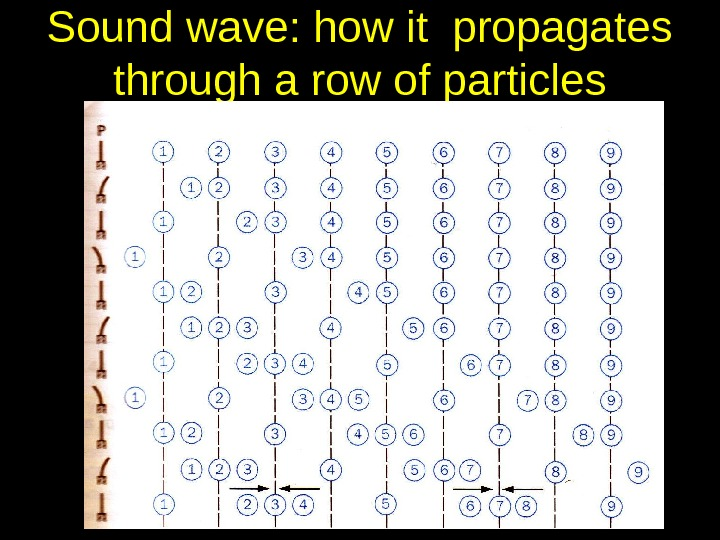Sound wave: how it propagates through a row of particles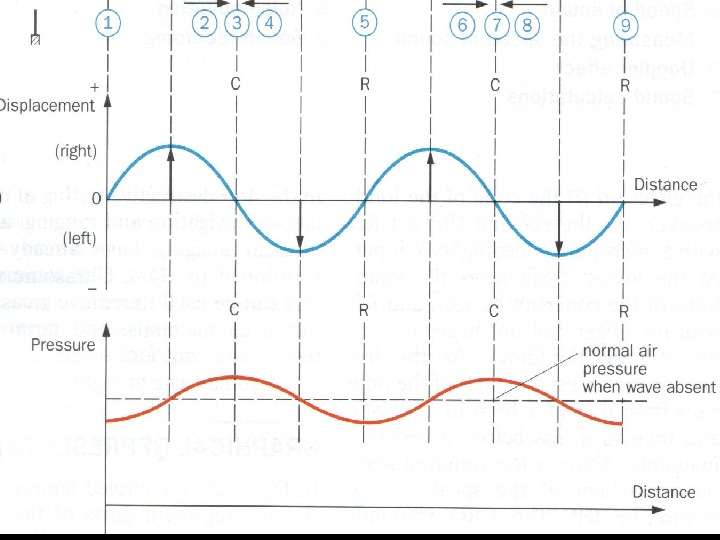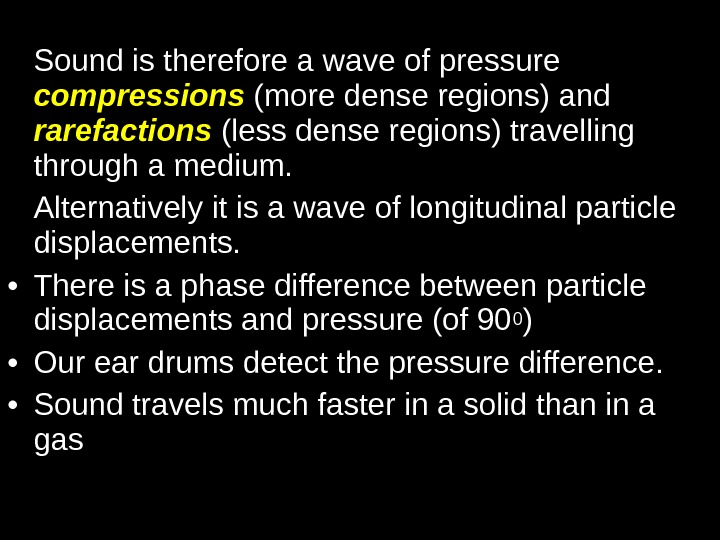Sound is therefore a wave of pressure compressions (more dense regions) and rarefactions (less dense regions) travelling through a medium. Alternatively it is a wave of longitudinal particle displacements. • There is a phase difference between particle displacements and pressure (of 90 0 ) • Our ear drums detect the pressure difference. • Sound travels much faster in a solid than in a gas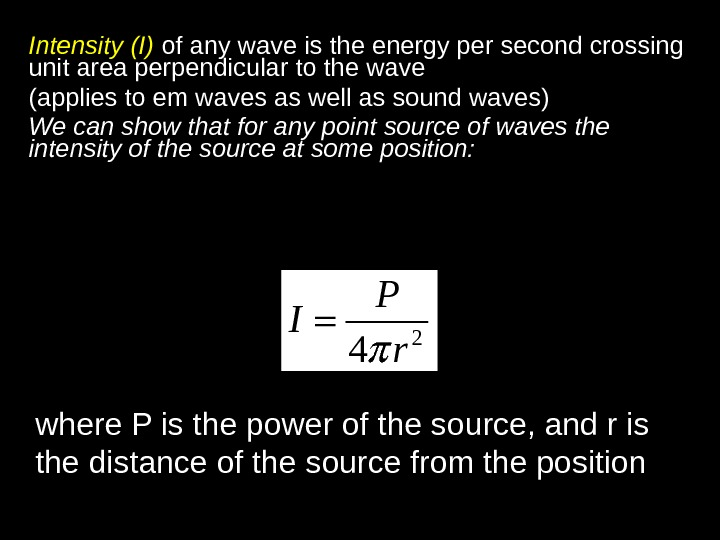Intensity (I) of any wave is the energy per second crossing unit area perpendicular to the wave (applies to em waves as well as sound waves) We can show that for any point source of waves the intensity of the source at some position: 2 4 P I r where P is the power of the source, and r is the distance of the source from the position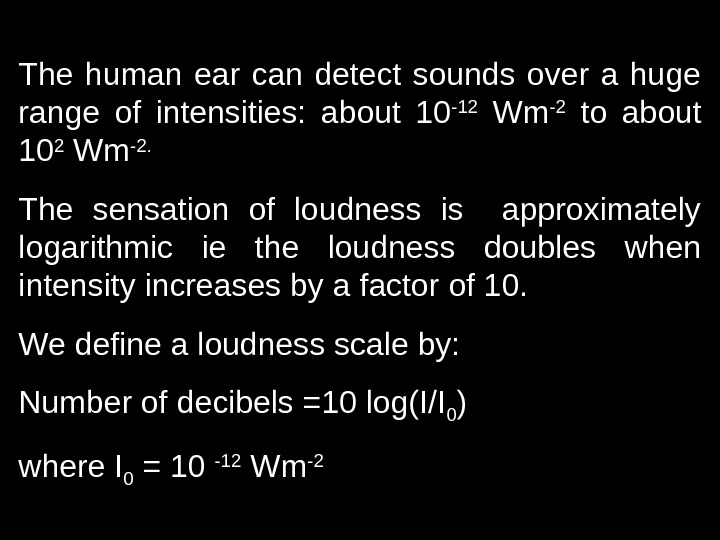The human ear can detect sounds over a huge range of intensities: about 10 -12 Wm -2 to about 10 2 Wm -2. The sensation of loudness is approximately logarithmic ie the loudness doubles when intensity increases by a factor of 10. We define a loudness scale by: Number of decibels =10 log(I/I 0 ) where I 0 = 10 -12 Wm —The intensity of electromagnetic radiation from the sun arriving at the collector panel of a solar water heater is 500 W m -2. How much energy does the heater receive in 1 hour if the area of the collector is 2. 5 m 2 ? Example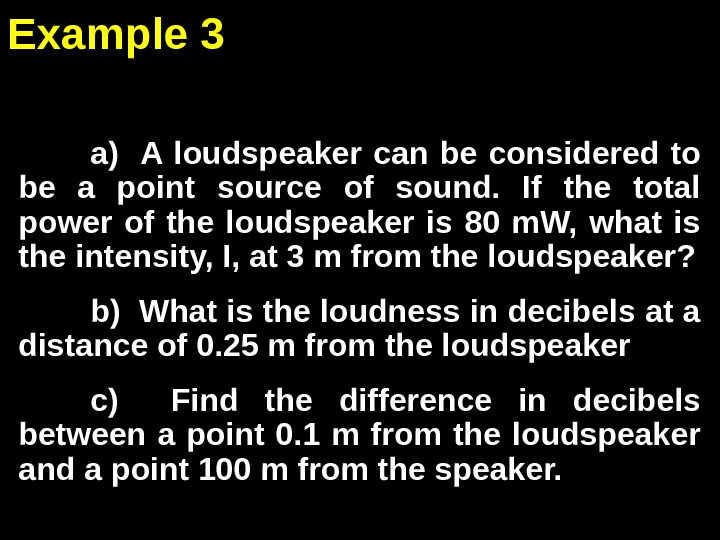a) A loudspeaker can be considered to be a point source of sound. If the total power of the loudspeaker is 80 m. W, what is the intensity, I, at 3 m from the loudspeaker? b) What is the loudness in decibels at a distance of 0. 25 m from the loudspeaker c) Find the difference in decibels between a point 0. 1 m from the loudspeaker and a point 100 m from the speaker. Example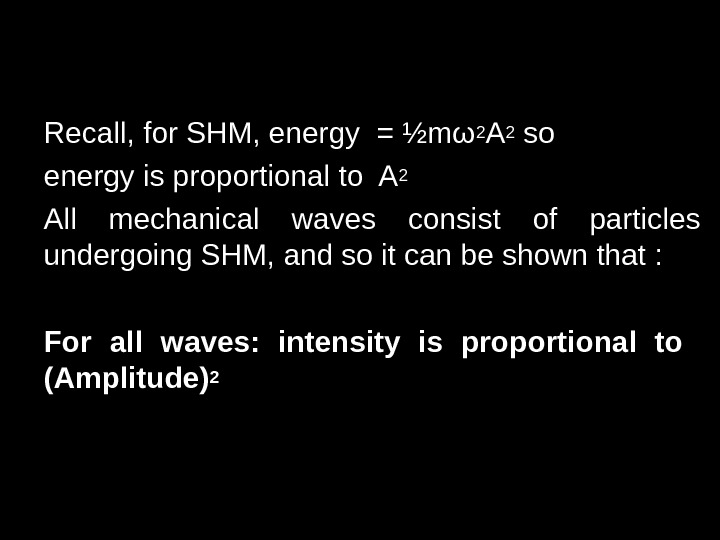Recall, for SHM, energy = ½mω 2 A 2 so energy is proportional to A 2 All mechanical waves consist of particles undergoing SHM, and so it can be shown that : For all waves: intensity is proportional to (Amplitude)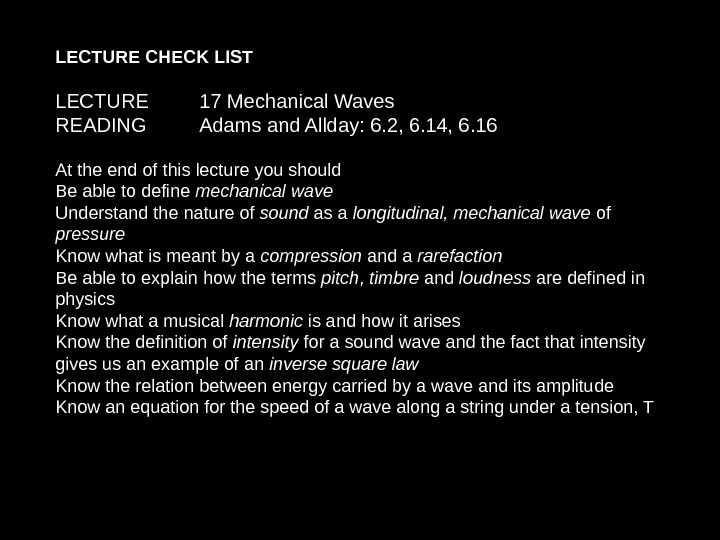LECTURE CHECK LIST LECTURE 17 Mechanical Waves READING Adams and Allday: 6. 2, 6. 14, 6. 16 At the end of this lecture you should Be able to define mechanical wave Understand the nature of sound as a longitudinal, mechanical wave of pressure Know what is meant by a compression and a rarefaction Be able to explain how the terms pitch , timbre and loudness are defined in physics Know what a musical harmonic is and how it arises Know the definition of intensity for a sound wave and the fact that intensity gives us an example of an inverse square law Know the relation between energy carried by a wave and its amplitude Know an equation for the speed of a wave along a string under a tension, T